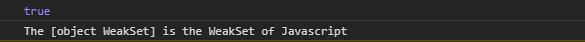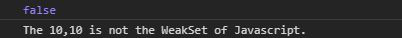# Underscore.js _.isWeakSet() Function

Underscore.js is a JavaScript library that makes operations on arrays, string, objects much easier and handy.
he _.isWeakSet() function is used to check whether the given object is JavaScript weakset or not. When linking the underscore.js CDN, the “_” is attached to the browser as global variable.

Syntax :

`_.isWeakSet( object );`

Parameters:

• object: It is any JavaScript object such as array, string, maps, set etc.

Return Value: It returns the boolean value. If the set is a weak set it returns true otherwise returns false.

Example 1: When a weak set is given it returns true.

 ` ` `<``html` `lang``=``"en"``> ` ` `  `<``head``> ` `    ``<``meta` `charset``=``"UTF-8"``> ` `    ``<``meta` `name``=``"viewport"` `content``= ` `        ``"width=device-width, initial-scale=1.0"``> ` ` `  `    ``<``script` `src``= ` `"https://cdnjs.cloudflare.com/ajax/libs/underscore.js/1.9.1/underscore-min.js"``> ` `    `` ` ` ` ` `  `<``body``> ` `    ``<``script``> ` ` `  `        ``// Creating a weak set using ` `        ``// constructor ` `        ``var obj = new WeakSet(); ` ` `  `        ``// Using the _.weakSet() function ` `        ``var isWeakSet = _.isWeakSet(obj); ` `        ``console.log(isWeakSet) ` ` `  `        ``// If the given object is weakset  ` `        ``// it prints the object is weak set. ` `        ``if (isWeakSet) ` `            ``console.log(`The \${obj} is the  ` `                    ``WeakSet of Javascript.`) ` `        ``else ` `            ``console.log(`The \${obj} is not  ` `                ``the WeakSet of Javascript.`) ` `    `` ` ` ` ` `  ` `

Output:Example 2: When an array is given the output is false.

 ` ` `<``html` `lang``=``"en"``> ` ` `  `<``head``> ` `    ``<``meta` `charset``=``"UTF-8"``> ` `    ``<``meta` `name``=``"viewport"` `content``= ` `        ``"width=device-width, initial-scale=1.0"``> ` ` `  `    ``<``script` `src``= ` `"https://cdnjs.cloudflare.com/ajax/libs/underscore.js/1.9.1/underscore-min.js"``> ` `    `` ` ` ` ` `  `<``body``> ` `    ``<``script``> ` ` `  `        ``// Creating a array of size  ` `        ``// 2 using constructor ` `        ``var obj = new Array(2); ` ` `  `        ``// Filling value 10in the array ` `        ``obj.fill(10) ` ` `  `        ``// Using the _.weakSet() function ` `        ``var isWeakSet = _.isWeakSet(obj); ` `        ``console.log(isWeakSet) ` ` `  `        ``// If the given object is weakset  ` `        ``// it prints the object is weak set ` `        ``if (isWeakSet) ` `            ``console.log(`The \${obj} is the ` `                ``WeakSet of Javascript.`) ` `        ``else ` `            ``console.log(`The \${obj} is not  ` `                ``the WeakSet of Javascript.`) ` `    `` ` ` ` ` `  ` `

Output:My Personal Notes arrow_drop_upCheck out this Author's contributed articles.

If you like GeeksforGeeks and would like to contribute, you can also write an article using contribute.geeksforgeeks.org or mail your article to contribute@geeksforgeeks.org. See your article appearing on the GeeksforGeeks main page and help other Geeks.

Please Improve this article if you find anything incorrect by clicking on the "Improve Article" button below.